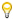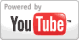Solving Polynomial Equations - Algebra Lesson
Grade: 7 - 12 | In this lesson, students learn that the first step in solving polynomial equations is to set the given equation equal to zero, the next step is to factor, and the final step is to set each of the resulting factors equal to zero and solve each equation.Add to My DashboardAlgebra
For Other Topics :Quiz Games by TopicInteractive DiagramsFlow Chart GamesVocabulary GamesSlide PuzzlesJigsaw PuzzlesCreate PresentationsBrain GamesVideos by Topic### Search Videos & Games